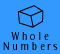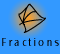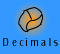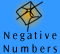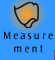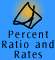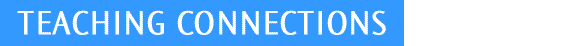| Introducing whole number arithmetic | Addition |
| Subtraction | Multiplication | Division |Learning Basic Facts | Estimation and Mental Computation

Introducing whole number arithmetic

The following key ideas must be emphasised:

 place value our number system has a base of ten the role of zero as a placeholder the additive property of place value (that 245 represents 200, 40 and 5 added) the multiplicative property of place value ( that the value of each successive column on the endless base ten chain is ten times the previous i.e. hundred = 10 x ten, thousand = 10 x hundred, ten thousand = 10 x thousand etc)

Place value

Although we have introduced place value using MAB, you would not do so with children. Use unstructured proportional materials first, e.g., bundling of icy pole sticks and straws before structured proportional materials such as MAB. Finally, use non-proportional models such as the abacus and counters.

Begin by making bundles of ten (icy pole sticks etc) to show the structure of numbers such as 23 from 2 bundles of ten and 3 more. In preparation for arithmetic algorithms, play lots of trading games. Make sure the activities include both the composition and decomposition of numbers, e.g., bundling (ones and tens) and unbundling (tens and ones). It is important to establish the notion that numbers can be represented in several ways, so that 245, for example, is 200 + 40 + 5 or 200 + 45 etc. Number expanders are excellent to demonstrate this and show possibilities.

Operations in general

The concepts of addition, subtraction, multiplication and division need to be discussed separately from the algorithms as well as with them. The algorithms are methods to carry out the specified calculation. There are also many other possible algorithms that are not usually taught in school, but which children with strong conceptual foundation may be able to invent for themselves.

Activities to develop the meaning of the operations should be based in real life examples. Use real life situations, stories, picture stories and word problems. Be aware there are valid different readings of picture stories. With word problems ensure that you use the full range of problem situations. For example, addition problems arise from situations of combining different sets together and they also arise from situations where one quantity is increased by a given amount. As another example, subtraction applies in situations where one quantity is taken away from another, but it also applies when a difference between two quantities has to be found.

Begin with the meaning(s) of the operation. This will need continued attention throughout the primary years. By understanding what the operation means, children will be able to develop basic facts and be able to deal with simple numbers. As this develops, they will also be able to "invent" ways of carrying out more complicated calculations. From these invented methods, standard algorithms can be introduced as a way of carrying out calculations more efficiently. Teaching each algorithm progresses through a variety of intermediate written forms, gradually working towards a quick and economically written form.

By the end of primary school, children should be efficient with all formal written algorithms for the 4 operations using reasonable numbers, but in this age of calculators, there is no need for excessive practice of lengthy calculations.

Estimation and mental calculation are addressed at every stage. Children can share their own methods and teachers can point out some useful techniques (e.g. building up to round numbers).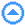Addition is introduced in the following sequence:

 Using the basic addition facts Adding 2 digit numbers — gradually refining an efficient written algorithm Adding 3 or more digit numbers NOTE: Estimation and mental addition is covered throughout the development of the topic

Children develop the basic addition and subtraction facts from counting. The basic addition and subtraction facts should be learnt together. There are many games which help children consolidate knowledge of basic facts.

Simple examples such as 3 + 6 come from counting skills before moving onto examples like 24 + 3 and then 24 + 8 where crossing of tens is encountered. At this stage a strong understanding of place value is needed. Recording can be in the form of informal jottings done either horizontally or vertically (labelling tens and ones).

Adding 2 digits is begun mentally in the early years of primary school and gradually extended so that children can use informal methods (mental or supported by jottings) in the middle primary years.

The formal algorithm can be introduced around Year 3 and will follow the understanding of place value and the meaning of the operation and be consolidated in the middle primary years. It should be demonstrated step by step, initially with place value materials. Labelled place value columns should be used as an intermediate stage. The role of the formal algorithm is to enable us to deal with more complicated additions and to provide a guaranteed short cut method to give us an answer for adding any whole numbers — it does not provide a means of understanding addition. It is important that understanding comes before practising the algorithm to efficiency.

Encourage children to do questions mentally and that will involve them being able to apply a wide variety of methods. Children should be using mental skills throughout primary school, including well chosen examples involving 3 and 4 digit calculations.

 primary stage types of examples methods used early years 3 + 6 24 + 3 mentally adding mentally adding middle years 399 + 15 (mentally) adding 2 and 3 digit numbers mentally adding informal methods of recording, written formal algorithm later years 395 + 427 (mentally) adding numbers of any number of digits mentally adding formal written algorithmSubtraction

Subtraction is introduced in the following sequence:

 Basic subtraction facts Decomposition algorithm - with gradual progression towards subtracting 2 digit numbers and 3 or more digit numbers - gradually refining an efficient written algorithm Alternative algorithms (if desired) NOTE: Estimation and mental subtraction is covered throughout the development of the topic

Children will already be familiar with the basic subtraction facts, learned in conjunction with basic addition facts. Stress the inverse relationship between addition and subtraction. There are many games that help children consolidate knowledge of basic facts.

The decomposition algorithm is usually introduced as the formal algorithm for subtraction since it has the strong advantage of being able to be modelled by concrete materials such as MAB. It should be demonstrated step by step using place value materials before being applied in an abstract way.

Later, some teachers like to teach the equal additions algorithm as an alternative as it is quicker and easier to use, particularly when dealing with more complicated subtractions involving larger numbers or when there are a lot of zeros in the minuend.

Teachers also need to be familiar with the major algorithms so they can cater for children coming from different backgrounds (e.g. other countries) who may already be using an alternative method.

Encourage Years 5 & 6 (Level 4) students to use a variety of mental strategies including complementary addition to solve subtraction problems.

As with all whole number operations it is important that the focus is on understanding the process of the operation and that students regularly tackle problems mentally. Once learnt, algorithms should be used by students when problems are too challenging to do mentally.Multiplication

Multiplication is introduced in the following sequence:

 Basic multiplication facts Multiplication by a single digit — gradually refining an efficient written algorithm Multiplication by a multiple of ten Multiplication by 2 or more digits — gradually refining an efficient written algorithm NOTE: Estimation and mental multiplication is covered throughout the development of the topic. Extending the meaning of multiplication beyond equal groups takes place mainly in the middle years of schooling.

Learning multiplication tables is often seen as a daunting task. It is important that children understand that there is not a huge array of multiplication facts that must be rote learnt. Encourage children to build up the multiplication facts from facts they already know and by using the commutative and associative laws. Knowledge of commutativity (but NOT by name!) enables a child to know 7 x 5 if they already know 5 by 7. Knowledge of associativity (but NOT by name) enables a child to know that the numbers in the 6 times table are just twice the numbers in the 3 times table.

There are many games that help students consolidate knowledge of basic facts.

From early multiplication onwards, form groups as rows and columns. Introduce arrays in the early years. Use a multiplication grid for introducing basic multiplication facts from early years also. Presenting the basic facts in a grid is a good way of displaying the commutative law of multiplication and for learning and connecting the basic division facts. The traditional tables charts show patterns within individual tables, but do not highlight patterns that occur between them.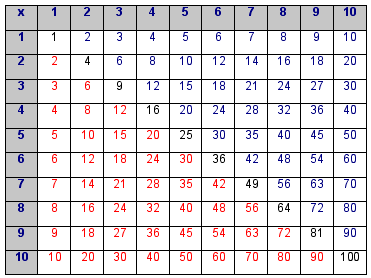multiplication grid

Multiplication is first introduced as repeated addition.

For example: 3 groups of 4 is 4 + 4 + 4.

Later, children can use this to help them learn basic facts. (Example: "I know 6 x 8 is 48. 7 x 8 is one more 8, so it is 48+8, that is 56")

Later children use repeated addition with the distributive law as in

3 x 15 = 15 + 15 + 15

or 3 x 15 = 3 x 10 + 3 x 5 = 30 + 15

The development of the algorithm proceeds through a number of stages moving towards a full understanding and the most efficient method.

Children must understand the intermediate stages of the algorithm.

We multiply by 10 by ‘adding zero’ (not a good phrase!) because this moves digits into the next place value column.

This idea then needs to be extended to multiplication by a multiple of 100 and by a multiple of 1000. A number slide demonstrates this well.

We multiply by a multiple of ten (such as 60) by first multiplying by 6 and then by 10.

Multiplication by a multi digit number occurs by combining the above, using the distributive law.

 32 6 0 x 4 1 3 6 0 ( 1 x 360) 1 4 4 0 0 (40 x 360) 1 4 7 6 0

Children need to understand what the partial products mean at each stage of the multiplication algorithm.

By the end of primary, children should be able to multiply any whole number by any whole number, in principle. In practice, very little time would be spent on (very) long multiplication because calculators are available.

There are a variety of alternative multiplication algorithms. For example, the "Lattice" multiplication algorithm can be used, particularly when children are having difficulty with place value.

The initial understanding of multiplication as repeated addition of equal groups needs to be extended to a variety of other situations in the middle years, so that multiplication and division by fractions and decimals is meaningful.Division

Division is introduced in the following sequence:

 Partition division meaning Quotition division meaning Basic division facts (inverse of multiplication) 2 digit division by a single digit with no remainders Remainders expressed as whole numbers, fractions and decimals Larger numbers, including a little work with divisors greater than 10 Division with remainders NOTE: Estimation and mental division is covered throughout the development of the topic, including division by multiples of 10, 100 etc. (e.g. 240 divided by 60)

The basic division facts can be learned in the context that they are the inverse of the multiplication facts.

3 division signs

There are 3 division signs that are commonly used. They are described in the table below.

 ÷ Rahn’s sign introduce division with this signlunar sign used for the division algorithm - or / vinculum this sign is used especially for fractions (i.e. 1 ÷ 9 = 1/9), in typed or electronic documents. and is also the form of division sign used in algebra.

Remainders

Start with whole number remainders and then move onto remainders expressed as common fractions and as decimal fractions. This is a common source of error for children, and teachers must give care to explaining the different forms of remainders clearly.Learning Basic Facts

Knowledge of the basic facts of the four operations and place value is the foundation for the algorithms. Thinking strategies for recalling basic facts in each operation are necessary. In one sense, there are almost 400 separate facts to learn, but some simple relationships reduce this number to just a few dozen. The most important ideas are of inverse operations, commutativity and associativity.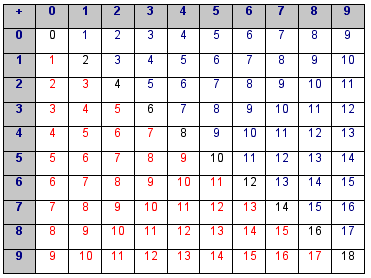The 100 basic facts for addition

Effective strategies used for establishing knowledge of basic facts of addition are,

counting on,

 Example 1: 6 and 1 more is 7 5 and 2 more is 7 (5, 6, 7)

doubles and near doubles,

 Example 2: 6 and 6 is 12 6 and 7 is 6 and 6 (12) and 1 more, so is 13

making to ten,

 Example 3: 8 and 3 (is 8 and 2 is 10 and 1 more) is 11 9 and 4 (is 9 and 1 is 10 and 3 more) is 13

Subtraction facts are linked as often as possible to the addition facts to exploit the inverse relationship,

 Example 4: 7 and 2 is 9 I have 7. How many more to make 9? Ans is 2 (missing addend 7,8,9 - stop at 9 and count how many steps) 9 take away 2 is 7 (counting back 9, 8, 7 - count two steps back) The difference between 9 and 7 is 2 (counting back of missing addend, 9,8,7 - stop at 7 and count how many steps)

Multiplication and division

By using arrays, strategies involving the distributive law (e.g. 6 by 8 is the same as 6 by 4 and 6 by 4) can be developed.

Example 1: 7 x 4 = 28, 7 groups of 4 stars = 28 stars7 groups of 4 stars is the same as 5 groups of 4 stars and 2 groups of 4 stars: 7 x 4 = 5 x 4 + 2 x 4 7 groups of 4 stars is the same as 4 groups of 7 stars: 7 x 4 = 4 x 7

Rotating the array can show the commutative law (e.g. 7 by 4 is the same as 4 by 7). Arrays can be used to show the inverse relationship between multiplication and division and so there is no need to learn a new set of basic facts for division.

Example 2: How many groups of 4 in 28?

We can see from the picture above that there are 7 groups of 4 in 28 as well as 4 groups of 7.

Example 3: If I have 28 stars and I want to share them equally among 7 people, how many stars will each person have?

We can also work out from the picture above that each person will get 4 stars.The 100 basic facts for multiplication

Estimation and mental computation

The skills of estimation and mental computation are built on the understanding of the basic concepts for each operation and use knowledge of basic facts, place value and rounding. Estimation and mental computation are needed to provide both approximate answers in many real life situations and to check reasonableness of written and calculator answers.

Estimation

Example 1: How many grains of rice in this 1 kilogram bag?

Some examples of the strategies children might use to estimate the answer to this problem include:

• a million grains of rice (because 1 million is a big number and there are a lot of grains of rice in the bag - this naive strategy needs improvement)
• I think I can hold about a thousand grains of rice in my hand and there looks like about 10 handfuls in that bag, so there are are 10000 grains of rice in the bag - this strategy can be refined by counting the number of grains in a handful, or half a handful.
• I know that there are 1000 grams in a kilogram and I think each grain of rice weighs a hundredth of a gram so there must be 1000 times 100 grains of rice in the bag which equals 100000 grains of rice. This strategy can be refined by weighing a convenient number of grains (e.g. 50 or 100 grains) so the estimate is based on firmer information.

Estimation also depends on children having a good sense of the size of units of measurement and knowing other "benchmark" data (e.g. a big pace is about 1 metre, my handspan is about 20 cm, a litre of water weighs a kilogram).